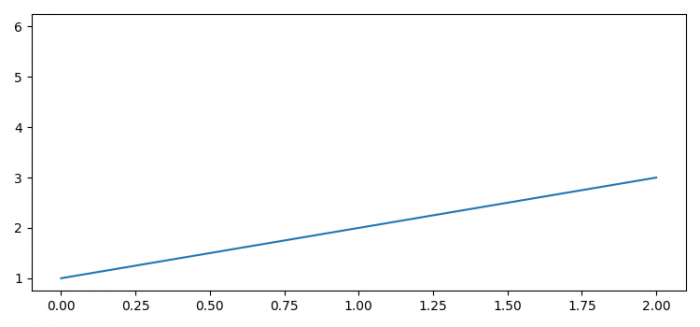# How to remove a specific line or curve in Matplotlib?

To remove a specific line or curve in Matplotlib, we can take the following steps −

• Set the figure size and adjust the padding between and around the subplots.
• Plot line1 and line2 using plot() method.
• Pop the second line and remove it.
• To display the figure, use show() method.

## Example

from matplotlib import pyplot as plt, image as mimg

plt.rcParams["figure.figsize"] = [7.50, 3.50]
plt.rcParams["figure.autolayout"] = True

line_1 = plt.plot([1, 2, 3])
line_2 = plt.plot([2, 4, 6])

line = line_2.pop(0)
line.remove()

plt.show()

## Output